• 状态空间描述为一种内部描述，为了状态空间对应的输出表达，在此探讨如何从状态空间表达式导出相应的传递函数表达式。 对于最一般形式的状态空间表达式： 其中：（u应为） 在初始条件为零的前提下，对上式作...

状态空间描述和控制理论传递函数之间存在什么关系呢？

状态空间描述为一种内部描述，为了状态空间对应的输出表达，在此探讨如何从状态空间表达式导出相应的传递函数表达式。

对于最一般形式的状态空间表达式：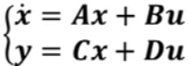其中：（u应为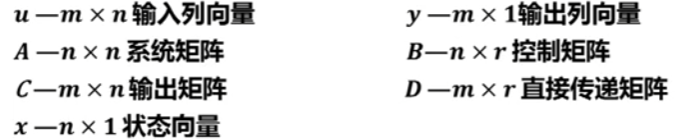在初始条件为零的前提下，对上式作拉氏变换，得：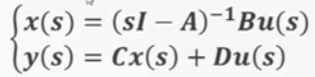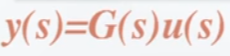得到系统的传递函数阵：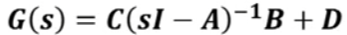其中，变换过程也非常简单，如下：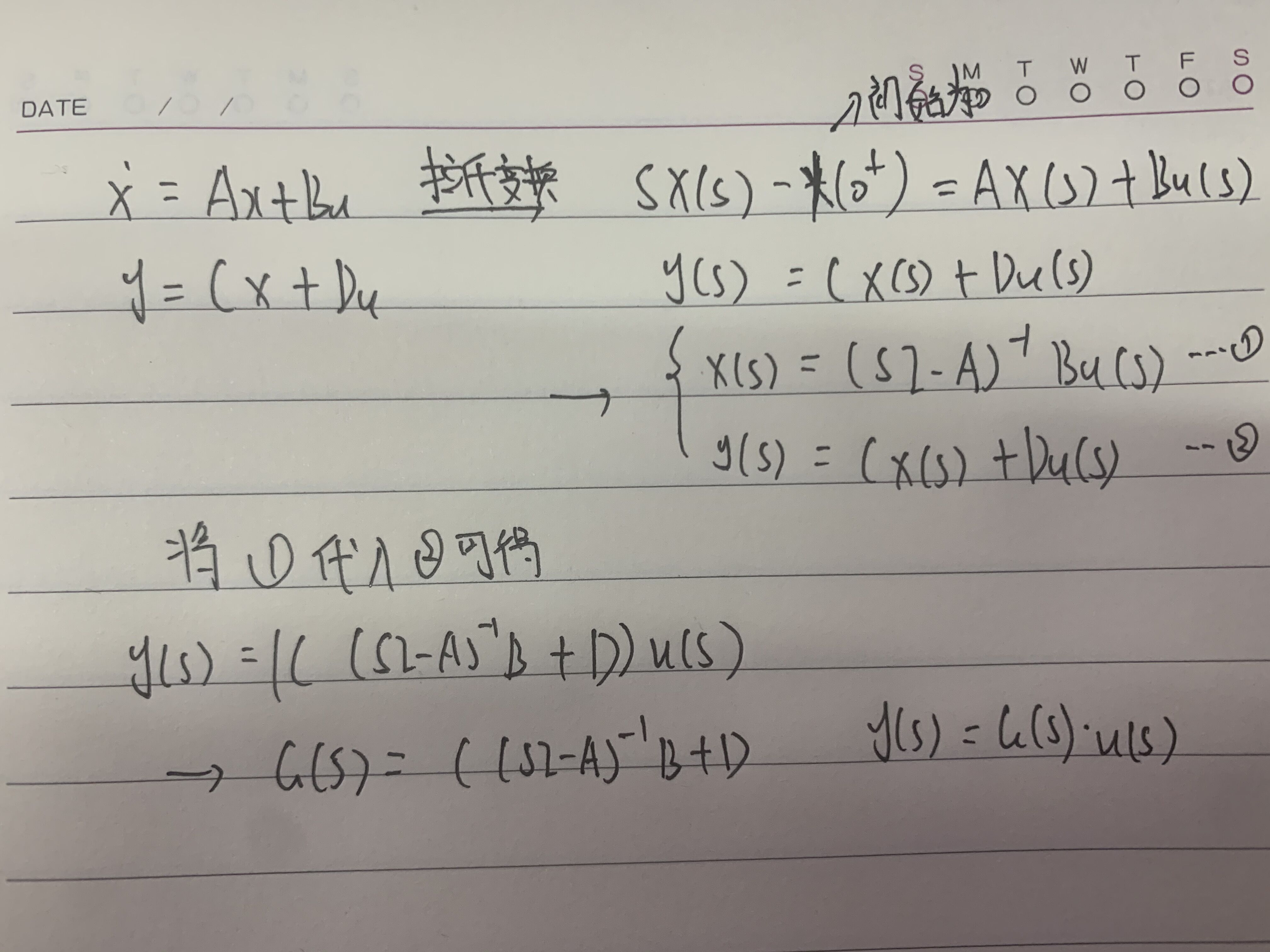系统的传递函数是一个的矩阵函数，设:为G(s)的元，则系统的传递函数阵可表示为以下的形式：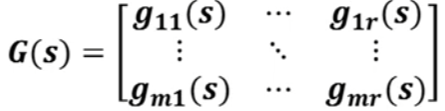容易看出，其元都是标量函数，它在物理上表示为第j个输入对第i个输出有相互关联。称为耦合关系，这正是多变量系统的特点。

以下考虑一个实例：

对于以下系统，求系统的传递函数阵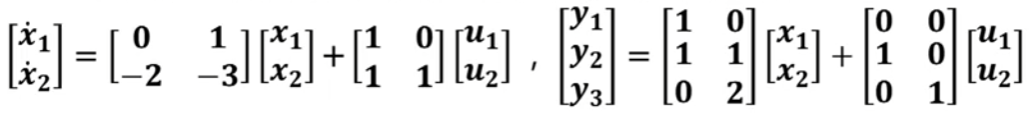解：由已知：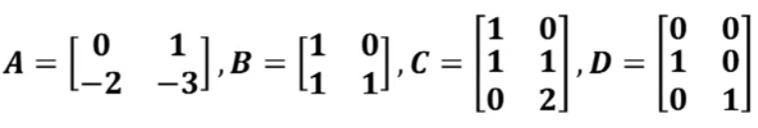，且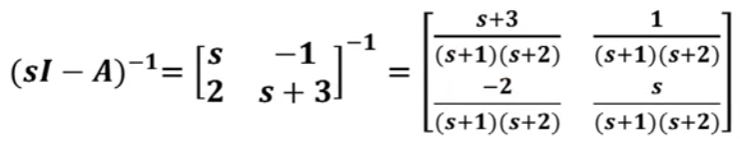（主对调，副变号）

所以，系统的传递矩阵为：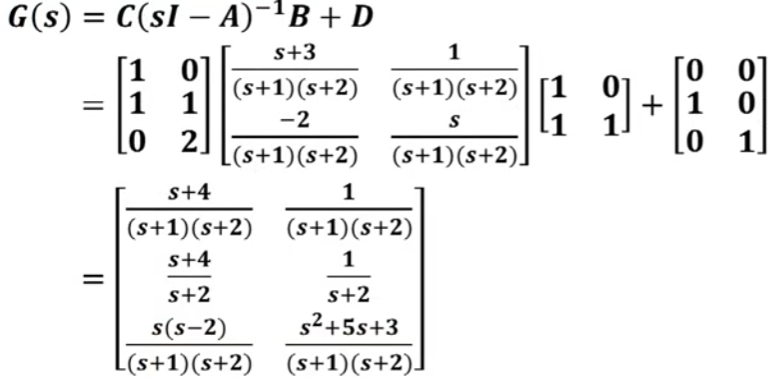展开全文• 该楼层疑似违规已被系统折叠隐藏此楼查看此楼这个对应的是单输入单输出的。多输入多输出的如下：% Thansfer ...% Step1：输入传递函数分子分母系数%From u1 to y1num1=[-0.0006086 -7.715e-005];den1=[1 1.079 0....

该楼层疑似违规已被系统折叠 隐藏此楼查看此楼

这个对应的是单输入单输出的。

多输入多输出的如下：

% Thansfer multiple transfer functions into sate space models

clc

clear all;

% Step1：输入传递函数分子分母系数

%From u1 to y1

num1=[-0.0006086 -7.715e-005];

den1=[1 1.079 0.2385 8.696e-005];

%From u2 to y1

num2=[0.03045 -0.0005537];

den2=[1 2.584 1.429 7.817e-005];

%From u1 to y2

num3=[3.135e-005 1.396e-007];

den3=[1 1.101 0.01934 5.657e-005];

%From u2 to y2

num4=[0.0003878 0.0001376];

den4=[1 0.6916 0.1158 0.003103];

% Step2：获得各单输入单输出状态空间模型

[A11,B11,C11,D11]=tf2ss(num1,den1);%From u1 to y1

[A21,B21,C21,D21]=tf2ss(num2,den2);%From u2 to y1

[A12,B12,C12,D12]=tf2ss(num3,den3);%From u1 to y2

[A22,B22,C22,D22]=tf2ss(num4,den4);%From u2 to y2

S_m11=ss(A11,B11,C11,D11);%From u1 to y1

S_m21=ss(A21,B21,C21,D21);%From u2 to y1

S_m12=ss(A12,B12,C12,D12);%From u1 to y2

S_m22=ss(A22,B22,C22,D22);%From u2 to y2

% Step3：按输入输出将状态空间模型写成矩阵形式，即为双输入双输出状态空间模型

P=[S_m11 S_m12;S_m21 S_m22];

% Step3：获得A，B，C，D矩阵

[A,B,C,D] = ssdata(P)

展开全文• 两种对二阶系统进行参数辨识的最小二乘算法，一种可以直接得到输入输出之间的传递函数，一种可以解出线性的差分方程各系数，再反解得到传递函数。附带的一个二阶系统的模型，可以自行下载导入输入输出数据进行试验。

一个具有两个输入和两个输出的模型：

模型结构如下图所示：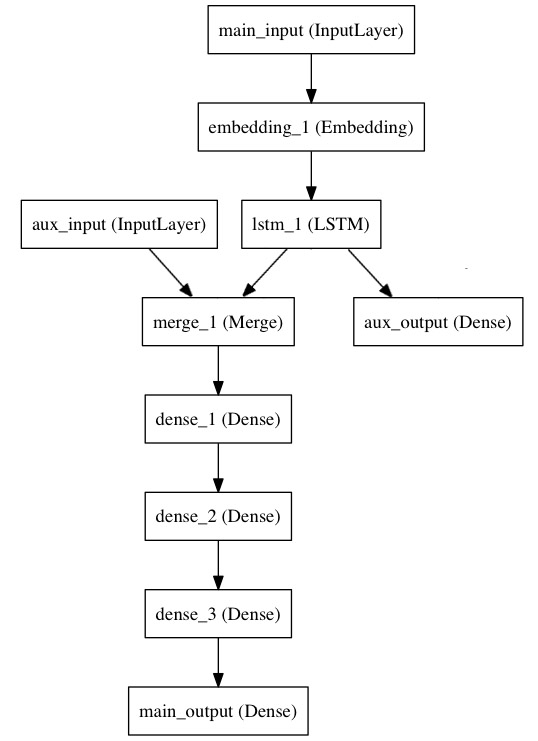主要输入接收新闻标题本身，即一个整数序列（每个整数编码一个词）。 这些整数在 1 到 10,000 之间（10,000 个词的词汇表），且序列长度为 100 个词。

from keras.layers import Input, Embedding, LSTM, Dense
from keras.models import Model

# 标题输入：接收一个含有 100 个整数的序列，每个整数在 1 到 10000 之间。
# 注意我们可以通过传递一个 "name" 参数来命名任何层。
main_input = Input(shape=(100,), dtype='int32', name='main_input')

# Embedding 层将输入序列编码为一个稠密向量的序列，
# 每个向量维度为 512。
x = Embedding(output_dim=512, input_dim=10000, input_length=100)(main_input)

# LSTM 层把向量序列转换成单个向量，
# 它包含整个序列的上下文信息
lstm_out = LSTM(32)(x)

在这里，我们插入辅助损失，使得即使在模型主损失很高的情况下，LSTM 层和 Embedding 层都能被平稳地训练。

auxiliary_output = Dense(1, activation='sigmoid', name='aux_output')(lstm_out)

此时，我们将辅助输入数据与 LSTM 层的输出连接起来，输入到模型中：

auxiliary_input = Input(shape=(5,), name='aux_input')
x = keras.layers.concatenate([lstm_out, auxiliary_input])

# 堆叠多个全连接网络层
x = Dense(64, activation='relu')(x)
x = Dense(64, activation='relu')(x)
x = Dense(64, activation='relu')(x)

# 最后添加主要的逻辑回归层
main_output = Dense(1, activation='sigmoid', name='main_output')(x)

然后定义一个具有两个输入和两个输出的模型：

model = Model(inputs=[main_input, auxiliary_input], outputs=[main_output, auxiliary_output])

现在编译模型，并给辅助损失分配一个 0.2 的权重。如果要为不同的输出指定不同的 loss_weights 或 loss，可以使用列表或字典。 在这里，我们给 loss 参数传递单个损失函数，这个损失将用于所有的输出。

model.compile(optimizer='rmsprop', loss='binary_crossentropy',
loss_weights=[1., 0.2])

我们可以通过传递输入数组和目标数组的列表来训练模型：

epochs=50, batch_size=32)

由于输入和输出均被命名了（在定义时传递了一个 name 参数），我们也可以通过以下方式编译模型：

model.compile(optimizer='rmsprop',
loss={'main_output': 'binary_crossentropy', 'aux_output': 'binary_crossentropy'},
loss_weights={'main_output': 1., 'aux_output': 0.2})

# 然后使用以下方式训练：
{'main_output': labels, 'aux_output': labels},
epochs=50, batch_size=32)

多输入单输出模型（共享网络层）

来考虑推特推文数据集。我们想要建立一个模型来分辨两条推文是否来自同一个人（例如，通过推文的相似性来对用户进行比较）。

实现这个目标的一种方法是建立一个模型，将两条推文编码成两个向量，连接向量，然后添加逻辑回归层；这将输出两条推文来自同一作者的概率。模型将接收一对对正负表示的推特数据。

由于这个问题是对称的，编码第一条推文的机制应该被完全重用来编码第二条推文（权重及其他全部）。这里我们使用一个共享的 LSTM 层来编码推文。

让我们使用函数式 API 来构建它。首先我们将一条推特转换为一个尺寸为 (280, 256) 的矩阵，即每条推特 280 字符，每个字符为 256 维的 one-hot 编码向量 （取 256 个常用字符）。

import keras
from keras.layers import Input, LSTM, Dense
from keras.models import Model

tweet_a = Input(shape=(280, 256))
tweet_b = Input(shape=(280, 256))

要在不同的输入上共享同一个层，只需实例化该层一次，然后根据需要传入你想要的输入即可：

# 这一层可以输入一个矩阵，并返回一个 64 维的向量
shared_lstm = LSTM(64)

# 当我们重用相同的图层实例多次，图层的权重也会被重用 (它其实就是同一层)
encoded_a = shared_lstm(tweet_a)
encoded_b = shared_lstm(tweet_b)

# 然后再连接两个向量：
merged_vector = keras.layers.concatenate([encoded_a, encoded_b], axis=-1)

# 再在上面添加一个逻辑回归层
predictions = Dense(1, activation='sigmoid')(merged_vector)

# 定义一个连接推特输入和预测的可训练的模型
model = Model(inputs=[tweet_a, tweet_b], outputs=predictions)

model.compile(optimizer='rmsprop',
loss='binary_crossentropy',
metrics=['accuracy'])
model.fit([data_a, data_b], labels, epochs=10)

层「节点」的概念

每当你在某个输入上调用一个层时，都将创建一个新的张量（层的输出），并且为该层添加一个「节点」，将输入张量连接到输出张量。当多次调用同一个图层时，该图层将拥有多个节点索引 (0, 1, 2…)。

在之前版本的 Keras 中，可以通过 layer.get_output() 来获得层实例的输出张量，或者通过 layer.output_shape 来获取其输出形状。现在你依然可以这么做（除了 get_output() 已经被 output 属性替代）。但是如果一个层与多个输入连接呢？

只要一个层仅仅连接到一个输入，就不会有困惑，.output 会返回层的唯一输出：

a = Input(shape=(280, 256))

lstm = LSTM(32)
encoded_a = lstm(a)

assert lstm.output == encoded_a

但是如果该层有多个输入，那就会出现问题：

a = Input(shape=(280, 256))
b = Input(shape=(280, 256))

lstm = LSTM(32)
encoded_a = lstm(a)
encoded_b = lstm(b)

lstm.output

>> AttributeError: Layer lstm_1 has multiple inbound nodes,
hence the notion of "layer output" is ill-defined.
Use get_output_at(node_index) instead.

好吧，通过下面的方法可以解决：

assert lstm.get_output_at(0) == encoded_a
assert lstm.get_output_at(1) == encoded_b

input_shape 和 output_shape 这两个属性也是如此：只要该层只有一个节点，或者只要所有节点具有相同的输入/输出尺寸，那么「层输出/输入尺寸」的概念就被很好地定义，并且将由 layer.output_shape / layer.input_shape 返回。但是比如说，如果将一个 Conv2D 层先应用于尺寸为 (32，32，3) 的输入，再应用于尺寸为 (64, 64, 3) 的输入，那么这个层就会有多个输入/输出尺寸，你将不得不通过指定它们所属节点的索引来获取它们：

a = Input(shape=(32, 32, 3))
b = Input(shape=(64, 64, 3))

conv = Conv2D(16, (3, 3), padding='same')
conved_a = conv(a)

# 到目前为止只有一个输入，以下可行：
assert conv.input_shape == (None, 32, 32, 3)

conved_b = conv(b)
# 现在 .input_shape 属性不可行，但是这样可以：
assert conv.get_input_shape_at(0) == (None, 32, 32, 3)
assert conv.get_input_shape_at(1) == (None, 64, 64, 3)

展开全文keras
• 传递函数的几种Matlab输入方式

千次阅读 多人点赞 2020-12-22 16:11:01
1：传递函数的几种Matlab输入方式 1.1、显示多项式的传递函数 matlab语句：分子分母的各项式系数按照降次排序分别计入num=[ ]，den=[ ]中，缺项系数补零。 传递函数 sys=tf(num,den) 1.2、因子形式的传递函数 ...

1：传递函数的几种Matlab输入方式

1.1、显示多项式的传递函数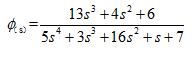matlab语句：分子分母的各项式系数按照降次排序分别计入num=[ ]，den=[ ]中，缺项系数补零。
传递函数 sys=tf(num,den)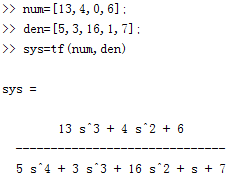1.2、因子形式的传递函数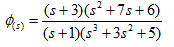matlab语句：利用conv（）实现两个多项式降次系数乘积运算。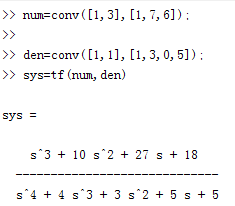1.3、显示零极点式传递函数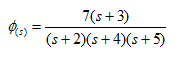matlab语句：zpk（）函数用法。z=[ ]输入零点 ，p=[ ]输入极点；k =[ ]输入增益。
sys=zpk（z,p,k）;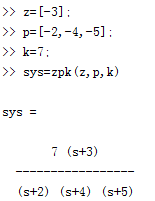1.4、直接传递函数输入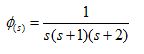matlab语句：s=tf(‘s’);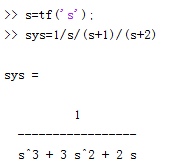2：传递函数多项式形式和零极点形式的转换

2.1、零极点形式转换为多项式形式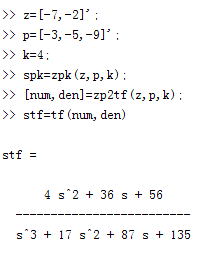2.2、多项式形式转换为零极点形式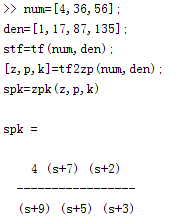展开全文• MATLAB的输入与输出函数

千次阅读 2019-08-29 10:19:58
输入以空格与换行符隔开的多行数值输出1. disp()(1)输出单个数值(2)输出一维数组(3)输出矩阵(4)输出字符串2. fprintf()(1)输出格式化的单个数值(2)输出格式化的一维数组(3)输出格式化的矩阵(4)输出格式化的字符串 ...MATLAB
• 变量 控制系统 传递函数 状态空间 模型转换 MATLAB变量系统传递函数模型转换为状态空间模型的MATLAB实现方法以下以双输入输出系统为例：% Thansfer multiple transfer functions into sate space models clc...
• 今天学习C++输入输出流的时候，遇到一个问题，先贴代码： void foutput (ofstream &fout, int m) { for (int i=1;i;i++) { fout *i+0.5; } } 上面这个函数的参数中用了一个ofstream类的变量，在下面...c++ 函数参数 文件输入输出流
• 控制系统的传递函数

千次阅读 2021-05-25 21:41:46
拉普拉斯变换是一种线性变换，所以传递函数只适用于描述线性定常系统，并且只适用于零初始条件情况下，对于非零初始条件可将初始条件看作输入使用。传递函数的几个性质如下： 1、传递函数是复变量s的有理分式，它...自动控制原理 典型环节 传递函数
• MATLAB 传递函数的相关函数

千次阅读 多人点赞 2019-06-17 23:15:57
1. 连续时间传递函数 1.1 多项式形式传递函数 num = 1; % 分子多项式的系数 den = [2, 3]; % 分母多项式的系数 G = tf(num, den) % 求传递函数 在matlab中，运行上述代码，可以得到传递函数 1.2 因式乘积形式...MATLAB 自动控制原理 现代控制理论 线性系统理论 传递函数
• matlab代码，直接应用输入输出数据辨识传递函数模型，且包含多种传递函数模型结构的辨识matlab建模
• 传递函数

千次阅读 2020-10-24 16:32:30
开环传递函数 G 闭环系统(上图) 闭环传递函数 T(s) = G/(1+G*H) 分母为 1+G*H，1+G*H=0的根即该闭环系统的极点，可用来判断闭环系统是否稳定。 特征根的实数为负，即极点都在s平面的左半平面，则系统稳定 开环传递...1024程序员节
• matlab画传递函数曲线

千次阅读 2021-05-07 07:26:34
如何matlab画开环传递函数的奈奎斯特图用MATLAB...稳定性可根据奈奎斯特判据判定：开环系怎样用MATLAB输入一个传递函数以G(s)=10/s(s+1)(s^2/4+1)为例这种传递函数是零极点描述形式,因而要使用sys=zpk(z,p,k)的命...
• 特别注意，G(s)在表现形式上和F(s)一样，都是关于s的函数，但其物理意义不一样：F(s)表示信号的数值变化规律，G(s)表示的是传递网络输入输出信号之间的数学计算关系，即输入信号是通过怎样的运算过程（数乘、积分、...
• 利用matlab由开环传递函数求闭环传递函数并求其单位冲击和阶跃响应例：设有一个系统的开环传递函数如下函数,并绘制输出阶跃响应曲线和脉冲响应曲线解：编程(见：\work\CT_tch\resp_2_20110522)clear all;close all;%...
• 自定义函数的输入与输出

千次阅读 2019-05-29 21:11:07
不一定非要使用C库中的标准函数，如果无法使用或者不想使用他们，完全可以在你GETCHAR（）和PUTCHAR（)的基础上自定义所需的函数，假设你需要一个类似put （），但是不会自动添加换行符的函数注意一下程序清单给出了...
• 格式化输入输出函数 一、printf、fprintf 和sprintf函数 函数原型： #include <stdio.h> int printf(const char *format, ...); int sprintf(char *s, const char *format, ...); int fprintf...
• Linux——shell脚本基础3：shell函数【参数传递输入输出】&内置函数   函数定义 1 退出状态 1 参数传递 2 标准IO 2 脚本调试 2 AND&OR 3 内置命令补充 3   函数定义 ...
• 金鸡一唱天下白：第三讲 控制系统的复域数学模型(传递函数)​zhuanlan.zhihu.com自动化人 - 知乎​www.zhihu.com在控制工程中，为了便于对系统进行分析...系统的结构图实质上是系统原理图与传递函数两者的综合。可以...
• 传递函数阶跃响应指标的matlab计算 对于一个常规的传递函数，我们在进行了阶跃仿真后经常需要给出对应的响应指标， sys = tf(1,[1 2 3]) y = step(sys) figure plot(y) 求解对应的阶跃指标结果 %% 求阶跃...传递函数 的matlab
• 许多教材和参考指南将运算放大器(运放)定义为可以执行各种功能或操作(如放大、加法和减法)的专用集成电路(IC)。...因此，运算放大器的输出通常以某种方式连接到输入，这种通常被称为电压反馈。在本...放大器的传递函数
• BP网络常用传递函数

万次阅读 2018-01-02 21:26:32
侵删 BP网络常用传递函数: BP网络的传递函数有多种。Log-sigmoid型函数的输入值可取任意值，输出值在0和1之间；tan-sigmod型传递函数tansig的输入值可取任意值，...线性传递函数purelin的输入输出值可取任意BP网络
• 传递函数：当初始条件为零时，系统输出量（响应函数）的拉氏变换表达式与系统输入量（驱动函数）的拉氏变换表达式之比，称为该系统的传递函数，其一般表达式为: 特征多项式：传递函数的分母为系统的特征多项式；...传递函数模型
• 可关注领取我的笔记pdf版哦~------------------------------------------------------------------------------我先对控制系统的数学模型进行简单的阐述，控制系统的数学模型是描述系统输入输出变量以及内部各变量...
• keras多输入多输出模型

千次阅读 2019-05-20 19:18:37
keras多输入多输出模型 主要输入(main_input): 新闻标题本身，即一系列词语。 辅助输入(aux_input): 接受额外的数据，例如新闻标题的发布时间等。 该模型将通过两个损失函数进行监督学习。 较早地在模型中使用主...
• 传递函数与状态空间

千次阅读 2019-09-21 23:31:37
传递函数与状态空间之间可相互转换，可以使用的matlab函数有 [A,B,C,D] = tf2ss(NUM,DEN) [NUM,DEN] = ss2tf(A,B,C,D,iu)    传递函数的形式唯一，但状态空间的形式不唯一，可以有多种。 1、一阶惯性...matlab 传递函数 状态空间 simulink
• MATLAB有一个常用的系统辨识工具箱，能够根据输入输出数据计算得到系统的传递函数，或是状态空间模型，下面给出具体的实施方法。 1、准备 将输入输出数据保存存到MATLAB的工作空间中； Matlab命令行窗口输入：...matlabmatlab simulink 二阶传递函数 时域...